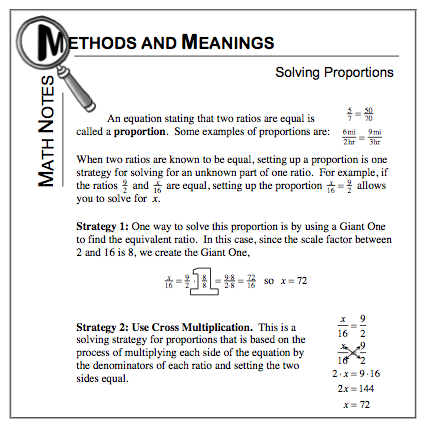### Home > MC1 > Chapter 10 > Lesson 10.1.3 > Problem10-39

10-39.

Solve each of the following proportions.

1. $\frac { 3 \text { minutes } } { 60 } = \frac { x } { 260 }$

2. $\frac { 5 \text { sodas } } { \ 1.30 } = \frac { 8 \text { sodas } } { C }$

3. $\frac { 4 \text { days } } { 5 \text { pizzas } } = \frac { x } { 35 \text { pizzas } }$

4. Write a word problem that could be solved by one of the proportions in parts (a) through (c).

For parts (a), (b), and (c), refer to the Math Notes box below.
Remember to show your work to avoid making unnecessary mistakes!In part (b), C is equal to $2.08$. Did you solve the proportion to parts (a) and (c)?

Hayden wrote a word problem for part (a) that read: If Rachel can type $60$ words in $3$ minutes, how many minutes will it take for her to type $360$ words? Does this sound like an appropriate word problem? Try writing your own!